# A fast and simple stretch-minimizing mesh parameterization

## Shin Yoshizawa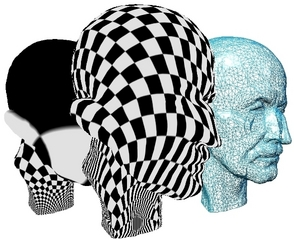In this research, we propose a fast and simple method for generating a low-stretch mesh parameterization. Given a triangle mesh, we start from the Floater shape preserving parameterization and then improve the parameterization gradually. At each improvement step, we optimize the parameterization generated at the previous step by minimizing a weighted quadratic energy where the weights are chosen in order to minimize the parameterization stretch. This optimization procedure does not generate triangle flips if the boundary of the parameter domain is a convex polygon. Moreover already the first optimization step produces a high-quality mesh parameterization. We compare our parameterization procedure with several state-of-art mesh parameterization methods and demonstrate its speed and high efficiency in parameterizing large and geometrically complex models.

This research is collaborated with Prof. Dr. A. G. Belyaev and Prof. Dr. H.-P. Seidel. The paper was published in Proc. Shape Modeling and Applications, pp. 200-208, Jun 7-11, 2004, Genova, Italy.

# C++ Code

You can download the C++ code which is TAR.GNUZIP file. After applied gunzip and tar -xf, please read README.txt and the following simple manual. Questions and Bug report are welcome to my e-mail: shin[_at_]riken.jp.

All rights are reserved by Shin Yoshizawa. This C++ source files are allowed for only a primary user for research and educational purposes. Don't use secondary use: copy, distribution, diversion, business purpose, and etc.. In no event shall the author be liable to any party for direct, indirect, special, incidental, or consequential damage arising out of the use of this program and source files. (*): The functions of the class PCBCGSolver are written in "Numerical Recipes in C++", The Art of Scientific Computing, William H. Press , Saul A. Teukolsky, William T. Vetterling, Brian P. Flannery. The copyrights of these functions of PCBCGSolver class are remained to them.

# Simple Manual

### How to use

• Compile: By using attached Makefile, you may run "make". You should use the later versions of g++ 2.95.
• Execute: Run "Parameterization input.ply2 output.ply2". Mesh data have to be constructed by the PLY2 format.
• Input (input.ply2): A disk topology 3D triangle mesh. I can not guarantee correct execution of the program for non 3-connected meshes for the unit square boundary. However you can try. In fact you can manage the boundary vertices of the non 3-connected triangle to assign to the conner vertex of the 2D parameter mesh by changing pickID in Polyhedron.h but it only solve the problem when such non 3-connected triangles are less than 4. Ex. the original mannequin head model (and all 1-to-4 subdivided models) has one such triangle.

•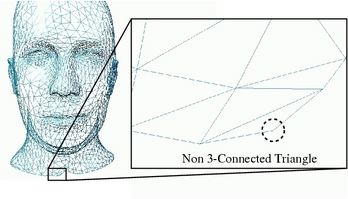• Output (output.ply2):A low-stretch parameter mesh whose z-coordinate is zero (in output PLY2 file, all z-coordinates are zero). Connectivity is equal to the input mesh. Therefore the parameterization (u,v) -f-> (x,y,z) can be defined by the piecewise linear mapping by using the barycentric coordinate of each triangles.

### Options

You can use the following options by changing the code in the constructor "Polyhedron()" of "Polyhedron.h". Also you should clean object file "make clean" then "make". The default setting is as follows:

Polyhedron(){
pickID=-1;
paramtype=2;
boundarytype=0;
weighttype=0;
iteNum=2000;
gammaP=1.0;
PCBCGerror=pow(0.1,6.0);
smooth=1;
intrinsiclambda=0.5;
}

• U^{1} or U^{Opt} (paramtype):Integer: You can choose U^{1} parameterization (after 1st step optimization):paramtype=1 or optimal parameterization paramtype=2 (default).

•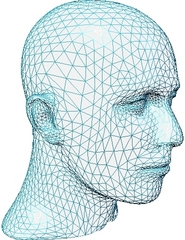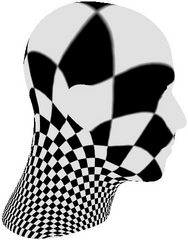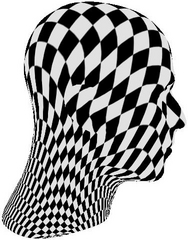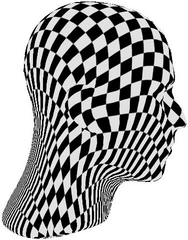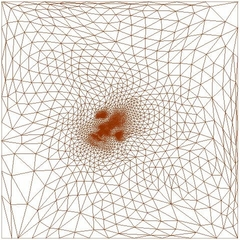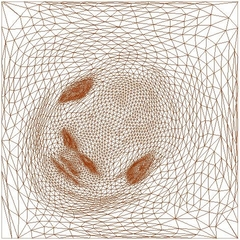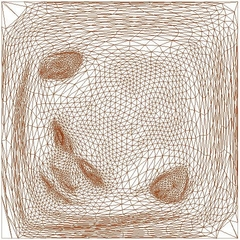---------Initial: Floater'97------------U^{1}--------------------U^{Opt}-
(*)these images are produced by setting the ID of boundary vertex of the non 3-connected triangle to pickID.

• Initial parameterization (weighttype):Integer: You can choose the following methods:
• weighttype=0; Shape Preserving (default): Floater CAGD 1997.
• weighttype=1; Tutte 1963.
• weighttype=2; Harmonic Map: Eck et al. SIGGRAPH 1995.
• weighttype=3; Intrinsic Map: Desbrun et al. EUROGRAPHICS 2002.
• blending parameter (intrinsiclambda):double: ((1-intrinsiclambda)*Chi + intrinsiclambda*Authalic). If intrinsiclambda=1 then it is equivalent to Eck's map. 0.5 is a default. 0<= intrinsiclambda<= 1.
• weighttype=4; Mean value: Floater CAGD 2003.
• Boundary Map (boundarytype):Integer: You can choose the following boundary polygons:
• boundarytype=0; unit square (default)
• boundarytype=1; unit circle
• boundarytype=2; natural boundary of the intrinsic parameterization
• Start point ID (pickID): Integer: You can set a ID of boundary vertex which will be a conner vertex when the boundary map is a unit square. If it is "-1" (default) the smallest number of boundary vertex ID will be the conner.
• Transfer Parameter (gammaP): double: In the paper we expressed "\eta". gammaP=1.0 is a default. 0< gammaP <=1. See the Figure 4 of the paper.
• Aggressive or Stable (smooth): If you will have a problem (instability) by using smooth=1 (default) then it is better to use smooth=2. However you should first try to change the parameters of the PCBCG (iteNum,PCBCGerror).
• PCBCG parameters: It is better to tune when you apply to large meshes.
• Maximum Iteration Number (iteNum): Integer: iteNum=2000 is a default setting. If you apply the program to the mesh which has more than 20000 vertices then you should increase the iteNum according to the vertex number of input mesh.
• Approximation Error Threshold PCBCGerror: double: 0.1^{6} is a default setting. When |Ax-b|/|b|<= PCBCGerror, linear solver would stop, see Numerical Recipes in C++.
• With-Without Boundary Stretch (boundarysigma): Integer: When we consider a metric tensor, it is not defined on the surface boundary. In discrete case, sometimes the boundary triangle stretches case the numerical instability such that some of them go to almost zero, -\inf or \inf. Therefore, this option allows us to ignore the boundary triangle stretches when we test the stretch distortion to decide whether stop the optimization or not. If boundarysigma is equal to 0 then we sum up the triangle stretches excepting boundary triangles. Otherwise, say boundarysigma=1, we sum up all triangle stretches. boundarysigma=1 is a default setting.

# Log

Mat 14, 2012: Fix a bug (initialization of inner vertices, no effect for UNIX/Linux) in Polyhedron.h for the latest Windows users, thanks to Xianyong Liu.
May 25, 2011: Fix a bug (initialization, no effect for UNIX/Linux) in IDList.h for the latest Windows users, thanks to Yuee Liu.
Apr. 12, 2011: Fix small memory leak in IDSet.cxx, thanks to Anuwat Dechvijankit.
Aug. 9, 2008: Set zero within initialization for non-GNU compilers, thanks to Mathieu Gauthier.
Apr. 18, 2008: Move to RIKEN.
Apr. 18, 2008: Solve g++ (GCC) version 4 problem.
Feb 16 2006: fix a boundary detection bug and add a boundary stretch neglect option.
Jul 19 2004: fix a natural boundary bug.